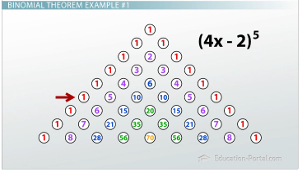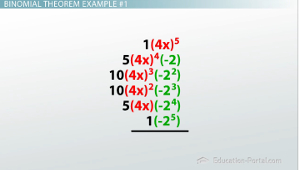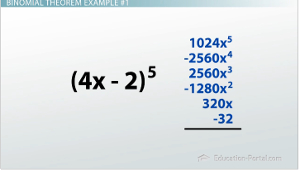# Binomial Theorem Practice Problems

An error occurred trying to load this video.

Try refreshing the page, or contact customer support.

Coming up next: What is a Mathematical Sequence?

### You're on a roll. Keep up the good work!

Replay
Your next lesson will play in 10 seconds
• 0:06 Steps of the Binomial Theorem
• 0:54 Binomial Theorem Example #1
• 4:26 Binomial Theorem…
• 6:20 Lesson Summary

Want to watch this again later?

Timeline
Autoplay
Autoplay
Speed

#### Recommended Lessons and Courses for You

Lesson Transcript
Instructor: Luke Winspur

Luke has taught high school algebra and geometry, college calculus, and has a master's degree in education.

The binomial theorem can be a really helpful shortcut, but it can also be really confusing. Brush up on your skills with this useful rule in these practice problems!

## Steps of the Binomial Theorem

Although the binomial theorem is the shortcut for raising a binomial to a power, it doesn't always feel that way. If you've found yourself getting confused while trying to use it, it can help to break it up into three steps.

Step 1: Find the corresponding row of Pascal's Triangle for your problem.

Step 2: Write decreasing powers of the first term, beginning with as many as we have in the problem.

Step 3: Write increasing powers of the second term, beginning with none of them.

I've found that if I try to do these steps all at once, or in different orders, I easily forget where I was or what I'd already done. Now I always stick to this order and write out a skeleton for my problem to keep track of which steps I've already done and which I still need to do.## Binomial Theorem Example #1

So let's go ahead and try that process with an example; maybe this example tells us to use the binomial theorem to expand (4x-2)^5.

Okay, step 1: which row of Pascal's Triangle do we need?

Well, let's jot down the triangle on the side of our paper here, remembering to add the two entries above to get the one below. You can either remember that we need to get the 6th row down (because the first row is the zero row), or you can just keep going until you see a 5 next to one of the 1s. Either way, we end up with the entries 1, 5, 10, 10, 5, 1.

I now go back over to the problem and copy these numbers down. This is what I call the 'skeleton' of the problem. It helps me keep my work organized and also prevents me from making a silly mistake.

Step 2 is to begin filling in the skeleton with decreasing powers of the first term. Because the binomial was originally being raised to the 5th power, I begin with a 4x^5. The next term gets a 4x^4, the next one a 4x^3, then 4x^2, then 4x^1, then finally 4x^0, or basically no 4xs at all.Step 3 is to do the opposite with the second term. We begin with zero -2s, but then gradually increase the number of them. Our next term has -2^1, then -2^2, then to the 3rd, to the 4th, and finally the last term has -2^5.

At this point our expression is essentially complete, but to be fully finished, we must follow the order of operations and first evaluate the exponents, then multiply together the resulting numbers in each term just to kind of have it simplified out.

The first term really only has one power to worry about, (4x)^5. 4^5 = 1,024 and x^5 is just x^5, so (4x)^5 is just 1,024x^5.

We again only have one power to worry about in the second term, so we evaluate it similarly and change (5)((4x)^4)(-2) to (5)(256x^4)(-2).

Our third term has two powers to be careful with, but the process is still basically the same. (4x)^3 turns into 64x^3, while -2^2 is 4. Continuing this process of evaluating exponents and re-writing for the remaining three terms would make our complete expansion look like so.

Now it is simply a matter of multiplying the numbers within each term. There's nothing to multiply except 1s in the first term, so it basically stays the same and is written as 1,024x^5.

We need to multiply a few things for the second term, though. 5, 256, and -2 are all there, so we multiply those together and we end up with -2,560x^4.

Continuing to multiply and re-write will give us our final answer as this. While it may be quite a bit of work, once you get the rhythm down it's really not too bad! Notice the alternating positive and negative coefficients. This is going to happen any time you have a negative second term, because it alternates from even to odd exponents. The odd ones leave it negative, but the even ones change it back to a positive number.To unlock this lesson you must be a Study.com Member.

### Register to view this lesson

Are you a student or a teacher?

### Unlock Your Education

#### See for yourself why 30 million people use Study.com

##### Become a Study.com member and start learning now.
Back
What teachers are saying about Study.com

### Earning College Credit

Did you know… We have over 160 college courses that prepare you to earn credit by exam that is accepted by over 1,500 colleges and universities. You can test out of the first two years of college and save thousands off your degree. Anyone can earn credit-by-exam regardless of age or education level.